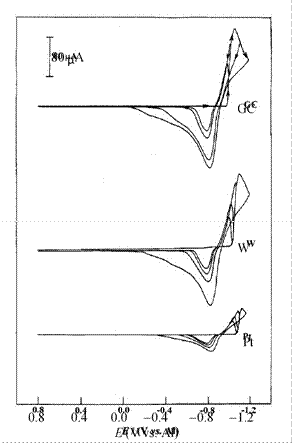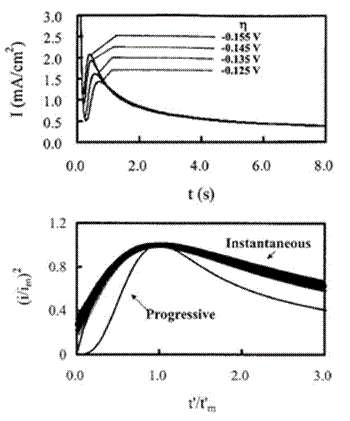# Electrochemistry of Room-Temperature/ Articles about substances designated as ionic liquids have begun to appear with increasing regularity in chemistry journals around the world, страница 16

The kinetic current, ik, can be estimated as a function of potential from the intercept of each plot of 1/i versus 1/ω1/2. This current leads to the potential dependent heterogeneous rate constant for the forward reaction, kf(E), via the following expression

ik = nFAkf (E)C*                                        (17)

Furthermore, k0 and αn can then be determined from the intercept and the slope of a plot of ln kf(E) vs. E E0', according to Eq.

(18):

0 αnF(E E 0' )

ln kf (E) = ln k +(18)

RT

Such a plot is illustrated in Fig. 9 for the data in Fig. 8. The formal potential, E0', can be estimated by the use of Eq. (15). But normally the diffusion coefficients for the oxidized and reduced forms of the solute are nearly the same, making E0' approximately equal to E1/2. From the data in Fig. 9, E0' was found to be -2.273 V, and k0 and αn were estimated to be 9.8 ± 0.8 × 10-5 cm s-1 and 0.36 ± 0.04, respectively.150

RRDE voltammetry has also been employed for electrochemical experiments in ionic liquids. It has been of great value in the analysis of plating reactions in chloroaluminate ionic liquids involving the underpotential deposition of aluminum-transition metal alloys.39,338 For the complete details of this procedure the reader should consult

the original literature.339

## (iii) Chronoamperometry

Chronoamperometry is the simplest controlled-potential technique available to the electrochemist and traces its roots to the seminal work of Frederick Gardner Cottrell in 1903.340 In spite of this simplicity, chronoamperometric techniques have been applied to a host of electrochemical problems in conventional molecular solvents and ionic liquids. Some of these uses include the measurement of diffusion coefficients, studies of metal nucleation, and the investigation of coupled homogeneous chemistry. This technique also forms the basis of a pulse voltammetric method for recording current-potential curves (see below).

The absolute magnitude of current obtained following a potential step of sufficient magnitude to deplete the electroactive species at the electrode surface in an unstirred solution, i.e., to produce a diffusion-controlled current, is given by the Cottrell equation,321

 nFAD1/2C*                               i(t) =1/2 1/2 (19)

π t

where t is the time (s) and all other symbols have their usual meaning. For a potentiostatic current-time transient resulting from simple, uncomplicated, diffusion-controlled reaction, i is proportional to t-1/2 or it1/2 is constant with t within the time limitations associated with the convective movement of the test solution caused by vibrations or thermal gradients. (The latter can be significant in

RTILs at elevated temperatures in poorly designed cells.) Thus, knowledge of it1/2 measured under diffusion-controlled conditions can be used along with Eq. (19) to calculate D.

The chronoamperometric technique has been employed extensively in RTILs and their higher temperature cousins to investigate nucleation/crystallization processes during the electrodeposition of metals on foreign substrates, e.g., substrates composed of materials different from the depositing metal. The presence of a nucleation overpotential can be readily determined by examining a cyclic voltammogram of the reaction of interest. For a nucleation-Figure 10. Cyclic staircase voltammograms recorded at different electrodes in mixtures of EtMeImCl + EtMeIm+BF4- containing 37.3 mmol L-1 Cd(II).  The sweep rates were 50 mV s-1. Reproduced from Ref. 341 with permission of Elsevier Ltd.